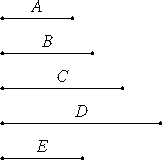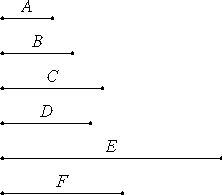# Proposition 36

To find the least number which three given numbers measure.

Let A, B, and C be the three given numbers.

It is required to find the least number which they measure.

VII.34

Take D the least number measured by the two numbers A and B.

Then C either measures, or does not measure, D.

First, let it measure it.But A and B also measure D, therefore A, B, and C measure D.

I say next that it is also the least that they measure.

If not, A, B, and C measure some number E less than D.

VII.35

Since A, B, and C measure E, therefore A and B measure E. Therefore the least number measured by A and B also measures E.

But D is the least number measured by A and B, therefore D measures E, the greater the less, which is impossible.

Therefore A, B, and C do not measure any number less than D. Therefore D is the least that A, B, and C measure.

Next, let C not measure D.

VII.34

Take E, the least number measured by C and D.

Since A and B measure D, and D measures E, therefore A and B also measure E. But C also measures E, therefore A, B, and C also measure E.I say next that it is also the least that they measure.

If not, A, B, and C measure some number F less than E.

VII.35

Since A, B, and C measure F, therefore A and B measure F. Therefore the least number measured by A and B also measures F. But D is the least number measured by A and B, therefore D measures F. But C also measures F, therefore D and C measure F, so that the least number measured by D and C also measures F.

But E is the least number measured by C and D, therefore E measures F, the greater the less, which is impossible.

Therefore A, B, and C do not measure any number which is less than E. Therefore E is the least that is measured by A, B, and C.

Q.E.D.

## Guide

The least common multiple of three numbers a, b, and c can be found as

LCM(a, b, c) = LCM(LCM(a, b), c)).

This proposition is used in the proof of proposition VII.39.